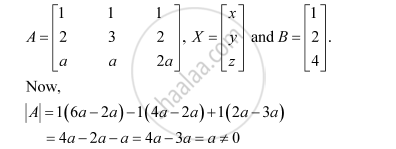Share

# Examine the Consistency of the System of Equations.X + Y + Z = 1 2x + 3y + 2z = 2 Ax + Ay + 2az = 4 - CBSE (Commerce) Class 12 - Mathematics

ConceptApplications of Determinants and Matrices

#### Question

Examine the consistency of the system of equations.

x + y z = 1

2x + 3y + 2z = 2

ax + ay + 2az = 4

#### Solution

The given system of equations is:

x + y z = 1

2x + 3y + 2z = 2

ax + ay + 2az = 4

This system of equations can be written in the form AX = B, where∴ A is non-singular.

Therefore, A−1 exists.

Hence, the given system of equations is consistent.

Is there an error in this question or solution?

#### Video TutorialsVIEW ALL 

Solution Examine the Consistency of the System of Equations.X + Y + Z = 1 2x + 3y + 2z = 2 Ax + Ay + 2az = 4 Concept: Applications of Determinants and Matrices.
S